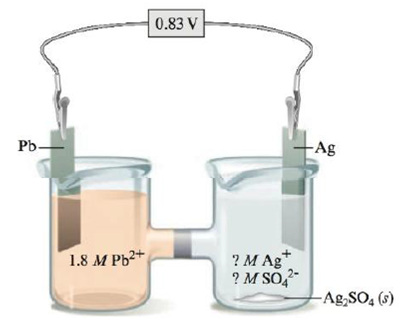# Consider the following galvanic cell: Calculate the K SP value for Ag 2 SO 4 ( s ). Note that to obtain silver ions in the right compartment (the cathode compartment), excess solid Ag 2 SO 4 was added and some of the salt dissolved.### Chemistry: An Atoms First Approach

2nd Edition
Steven S. Zumdahl + 1 other
Publisher: Cengage Learning
ISBN: 9781305079243

#### Solutions

Chapter
Section### Chemistry: An Atoms First Approach

2nd Edition
Steven S. Zumdahl + 1 other
Publisher: Cengage Learning
ISBN: 9781305079243
Chapter 17, Problem 143CP
Textbook Problem
54 views

## Consider the following galvanic cell:Calculate the KSP value for Ag2SO4(s). Note that to obtain silver ions in the right compartment (the cathode compartment), excess solid Ag2SO4 was added and some of the salt dissolved.

Interpretation Introduction

Interpretation:

The set up of a galvanic cell, in whose cathode compartment excess solid Ag2SO4 was added to obtain the silver ions. The Ksp value for Ag2SO4 has to be calculated.

Concept introduction:

The relationship between reduction potential and standard reduction potential value and activities of species present in an electrochemical cell at a given temperature is given by the Nernst equation.

The value of Ecell is calculated using Nernst formula,

E=E°(RTnF)ln(Q)

At room temperature, the above equation specifies as,

E=E°(0.0591n)log(Q)

Solubility product is applied only for those ionic compounds that are sparingly soluble. The product of solubility of ions is called solubility product and solubility is present in moles per liter.

To determine: The Ksp value of Ag2SO4 .

### Explanation of Solution

Given,

The concentration of Pb2+ is 1.8M

The cell reaction is,

Pb(s)+2Ag+(aq)Pb2+(aq)+2Ag(s)

The reaction taking place at cathode is,

Ag+(aq)+eAg(s)E°red=0.80V

The reaction taking place at anode is,

Pb(aq)Pb2+(aq)+2eE°ox=0.13V

Multiply reduction half reaction with the coefficient of 2 and add both the reduction and oxidation half-reaction,

2Ag+(aq)+2e2Ag(s)Pb(aq)Pb2+(aq)+2e

The final equation is,

Pb(s)+2Ag+(aq)Pb2+(aq)+2Ag(s)

The overall cell potential is calculated as,

E°cell=E°ox+E°red

Where,

• E°ox is the oxidation potential of the electrode.
• E°red is the reduction potential of the electrode.

Substitute the value of E°ox and E°red in the above equation as,

E°cell=E°ox+E°red=0.13V+0.80V=0.93V

The reaction involves the transfer of 2 moles of electrons.

The cell potential is calculated using the Nernst equation,

E=E°(0.0591n)log(Q)

Where,

E is the cell potential.

• E° is the cell potential at the standard conditions.
• Q is the activity of the species in the cell.
• n is the number of electrons involved in the reaction.

Substitute the values of Eo , n and Q in the above equation as,

E=0.93(0.05912)log([Pb2+][Ag+]2)0.83=0.930.02955log([1.8M][Ag+]2)3.39=log([1.8M][Ag+]2)

The equation is further simplified as,

3.39=log([1.8M][Ag+]2)3

### Still sussing out bartleby?

Check out a sample textbook solution.

See a sample solution

#### The Solution to Your Study Problems

Bartleby provides explanations to thousands of textbook problems written by our experts, many with advanced degrees!

Get Started

Find more solutions based on key concepts
Fried banana or vegetable snack chips make a healthy everyday snack choice for vegetarians. T F

Nutrition: Concepts and Controversies - Standalone book (MindTap Course List)

What are sister chromatids?

Human Heredity: Principles and Issues (MindTap Course List)

List three reasons a breastfeeding mother may develop mastitis.

Nutrition Through the Life Cycle (MindTap Course List)

A pendulum consists of a small object called a bob hanging from a light cord of fixed length, with the top end ...

Physics for Scientists and Engineers, Technology Update (No access codes included)

What is the tragedy implicit in Hardins The Tragedy of the Commons?

Oceanography: An Invitation To Marine Science, Loose-leaf Versin